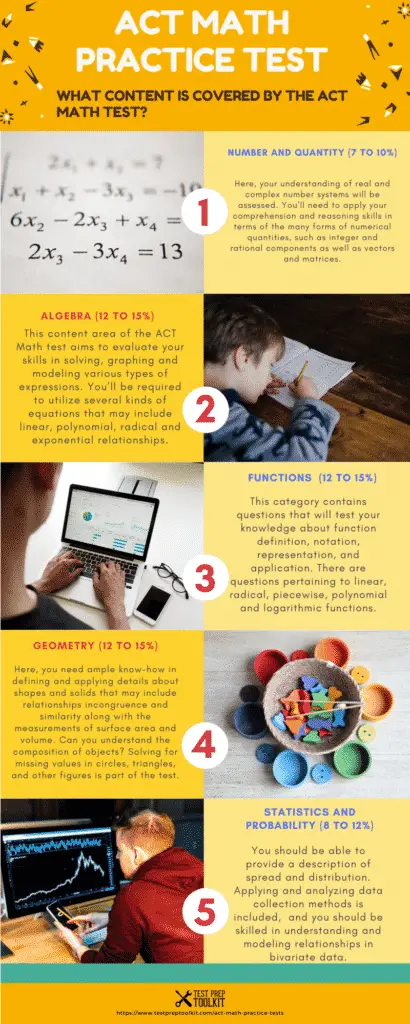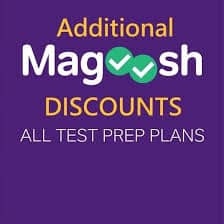# ACT Math Practice Test – Free Math Practice Questions

ACT Mathematics Practice tests to help you understand the original ACT Math tests you face during the exam. You will receive detailed scoring results at the end of each ACT Mathematics practice test to help you know your strengths and weaknesses.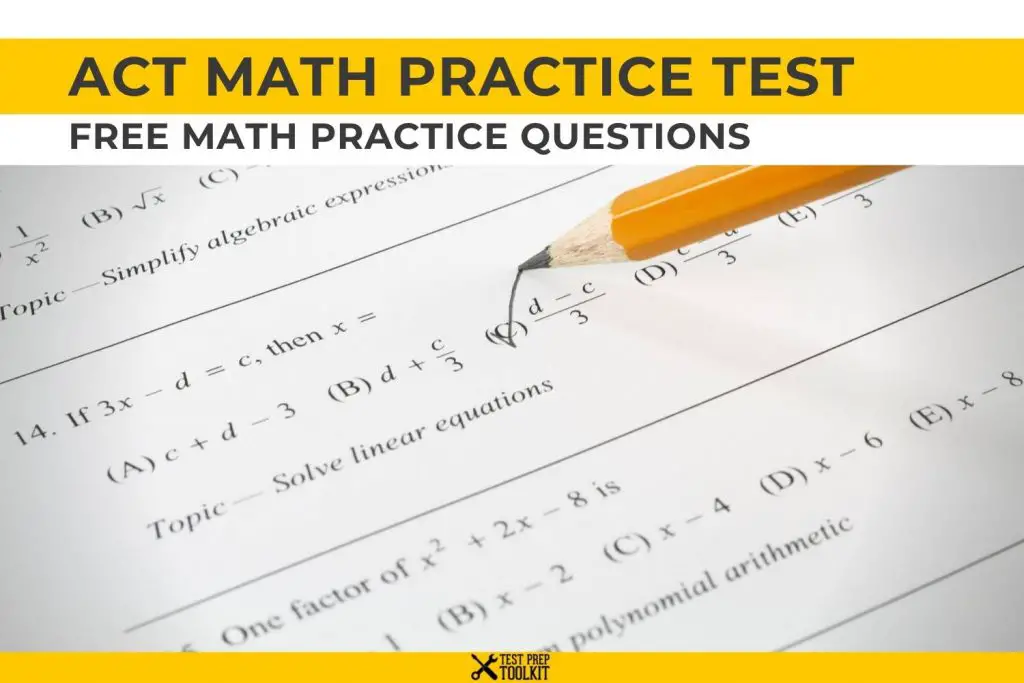DISCLOSURE: This post may contain affiliate links, meaning when you click the links and make a purchase, we receive a commission.

The ACT Math test is comprised of 60 test questions that have to be accomplished in 60 minutes. Test items in the ACT Mathematics test have been designed to assess students’ mathematical skills- particularly those that they have taken up in courses at the beginning of the 12th grade.

## No More Math Anxiety! Be One Step Ahead To Ace The ACT Math Test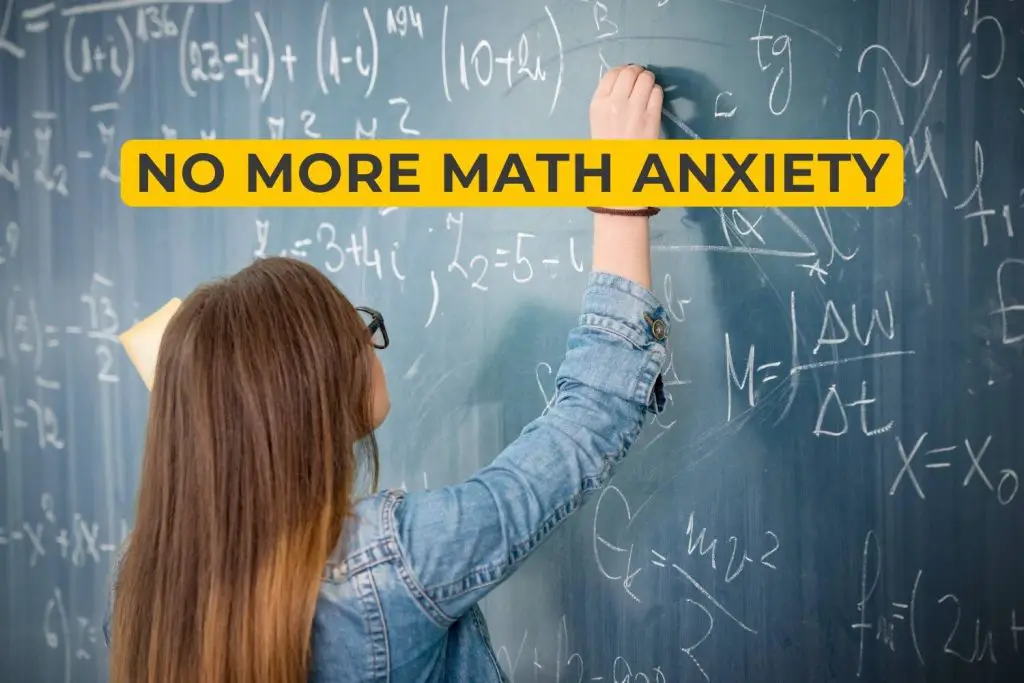The only thing to fear about Mathematics is ignorance. Math in itself as a subject doesn’t have to be tricky. You just have to know the proper approach to tackling its pertinent problems. Don’t let your Math anxiety get the better of you. The first thing that you have to do when commencing on your ACT Math test prep is to pacify your anxieties.

Don’t leave your ACT Mathematics test to chance. Your best bet by far to ace the ACT test is to sufficiently prepare. Realize that you can proficient in Math with the right tools and resources, along with your motivation to learn.

One of the most effectual ways to study for the ACT Mathematics test is to take practice  exams. In fact, studies have cited that 30% of a good study program is comprised of practice exams. Getting hold of effective ACT Mathematics practice exams makes you one step ahead of the game. Why? Because there are numerous astounding benefits of taking ACT Math practice tests. Among them is that it boosts your test-taking skills, knowledge, and confidence. It will make you aware of what to expect in the real ACT Mathematics test.

Check our ACT Practice Test – Full-Length Practice Questions with Explanation

Pick An ACT Mathematics Practice Test Here (Links to TPT ACT Math Practice Tests)

## What’s In The ACT Math Test?

### Here is a brief description: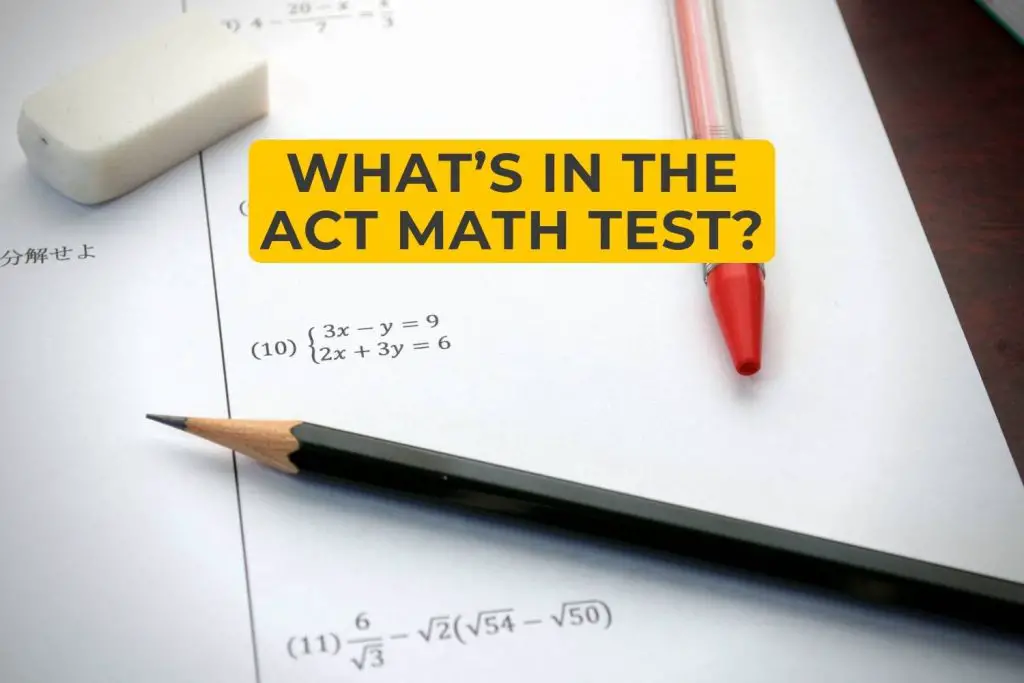The ACT Mathematics Test is a multiple-choice test that requires you to apply reasoning skills in solving practical Math problems. The majority of the questions in this section are self-contained while some may be related to specific sets of questions, such as a cluster of items pertaining to the same chart or graph. You don’t need to be able to recall complex mathematical formulas or compute equations extensively. However, you need to have ample know-how of basic formulas and computational skills. These will be utilized as a background for the problems.

The Math test covers material that emphasizes salient content parts that are necessary for proficient performance in entry-level math courses in college. There are 9 scores that are reported from the ACT Math test- one which is a total test score derived from the entire 60 questions, along with 8 category scores obtained from particular mathematical skills and knowledge.

Learn more: ACT Math Test Overview, Content-Type And Tips To Score High

## What Content Is Covered By The ACT Math Test?## Preparing for Higher Math (comprises 57 to 60% of the test)

• Number and Quantity (7 to 10%)

Here, your understanding of real and complex number systems will be assessed. You’ll need to apply your comprehension and reasoning skills in terms of the many forms of numerical quantities, such as integer and rational components as well as vectors and matrices.

• Algebra (12 to 15%)

This content part of the ACT Mathematics test aims to evaluate your skills in solving, graphing and modeling various types of expressions. You will be required to utilize several kinds of equations that may include linear, polynomial, radical and exponential relationships. Can you figure out the solutions to systems of equations although they may be signified by simple matrices? You will need to apply your know-how to applications and such.

• Functions  (12 to 15%)

This category contains questions that will test your knowledge about function definition, notation, representation, and application. There are questions pertaining to linear, radical, piecewise, polynomial and logarithmic functions. How skilled are you in manipulating and translating functions? Make ready as well in finding and applying the significant aspects of graphs.

• Geometry (12 to 15%)

Here, you need ample know-how in defining and applying details about shapes and solids that may include relationships incongruence and similarity along with the measurements of surface area and volume. Can you understand the composition of objects? Solving for missing values in circles, triangles, and other figures is part of the test. You will need to apply trigonometric ratios and equations of conic sections.

• Statistics and Probability (8 to 12%)

In this category, you should be able to provide a description of spread and distribution. Applying and analyzing data collection methods is included,  and you should be skilled in understanding and modeling relationships in bivariate data. Your knowledge in calculating probabilities such as related sample spaces will be tested.

## Integrating Essential Skills (Comprises 40 to 43% of  the Test)

The questions in this content part relate to concepts that students have typically learned prior to Grade 8. These include rates and percentages, area, surface area, volume, average and median and proportional relationships. Knowledge in number expression in different ways is required. You will be tackling problem-solving items of increasing complexity along with combining skills in longer chains of steps. Can you employ skills in various contexts and understand additional connections? Your skills in fluency will be assessed.

Related Topic: ACT Math Practice Tests 3

## Modeling (Comprises Approximately 25% of  the Test)

In this section, you are required to answer test items that pertain to the production, interpretation, understanding, evaluation, and improvement of models. On the overall, this category measures your proficiency in using modeling skills in relation to various topics in Math.